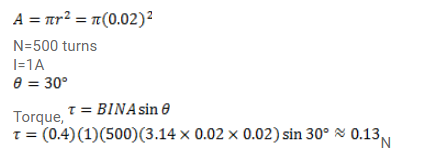# A circular coil of radius 2.0cm has 500 turns in it and carries a current of 1.0A.

Question:

A circular coil of radius $2.0 \mathrm{~cm}$ has 500 turns in it and carries a current of $1.0 \mathrm{~A}$. Its axis makes an angle of $30^{\circ}$ with the uniform magnetic field of magnitude $0.40 T$ that exists in the space. Find the torque acting on the coil.

Solution: